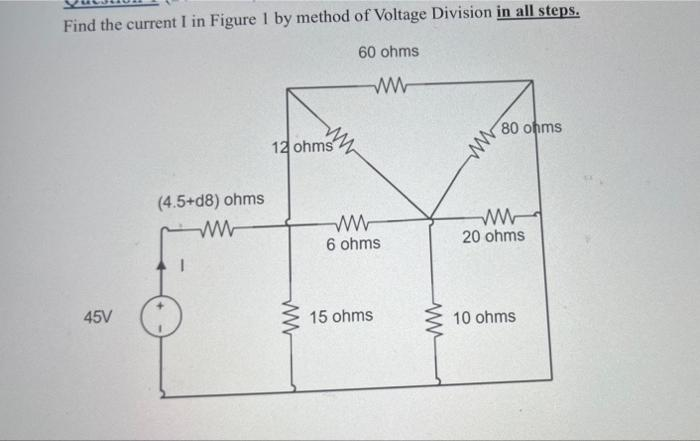# Question Find the current $$\mathrm{I}$$ in Figure 1 by method of Voltage Division in all steps.Transcribed Image Text: Find the current $$\mathrm{I}$$ in Figure 1 by method of Voltage Division in all steps.
Transcribed Image Text: Find the current $$\mathrm{I}$$ in Figure 1 by method of Voltage Division in all steps.